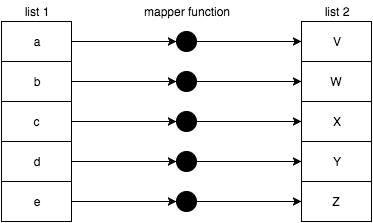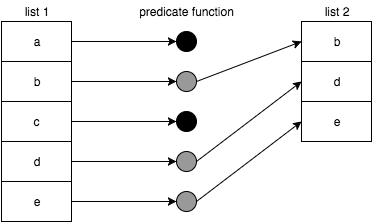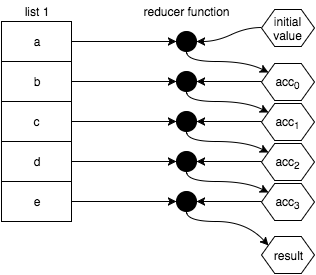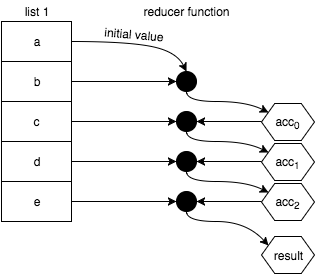# 第 8 章：列表操作

## 非函数式编程列表处理

• `forEach(..)`
• `some(..)`
• `every(..)`

`forEach(..)` 是遍历辅助函数，但是它被设计为带有副作用的函数来处理每次遍历；你或许已经猜测到了它为什么不是我们正在讨论的函数式编程列表操作！

`some(..)``every(..)` 鼓励使用纯函数（具体来说，就像 `filter(..)` 这样的谓词函数），但是它们不可避免地将列表化简为 `true``false` 的值，本质上就像搜索和匹配。这两个实用函数和我们期望采用函数式编程来组织代码相匹配，因此，这里我们将跳过它们。

## 映射

``````var x = 2, y;

// 转换／投影
y = x * 3;

// 变换／重新赋值
x = x * 3;
``````

``````var multipleBy3 = v => v * 3;

var x = 2, y;

// 转换／投影
y = multiplyBy3( x );
````````````function map(mapperFn,arr) {
var newList = [];

for (let idx = 0; idx < arr.length; idx++) {
newList.push(
mapperFn( arr[idx], idx, arr )
);
}

return newList;
}
``````

`mapperFn(..)` 自然地将传入的列表项做映射／转换，并且也传入了 `idx``arr`。这样做，可以和内置的数组的 `map(..)` 保持一致。在某些情况下，这些额外的参数非常有用。

``````map( ["1","2","3"], unary( parseInt ) );
// [1,2,3]
``````

Javascript 提供了内置的数组操作方法 `map(..)`，这个方法使得列表中的链式操作更为便利。

``````var one = () => 1;
var two = () => 2;
var three = () => 3;

[one,two,three].map( fn => fn() );
// [1,2,3]
``````

``````var increment = v => ++v;
var decrement = v => --v;
var square = v => v * v;

var double = v => v * 2;

[increment,decrement,square]
.map( fn => compose( fn, double ) )
.map( fn => fn( 3 ) );
// [7,5,36]
``````

### 同步 vs 异步

``````var newArr = arr.map();

``````

### 映射 vs 遍历

``````[1,2,3,4,5]
.map( function mapperFn(v){
console.log( v );            // 副作用!
return v;
} )
..
``````

### 一个词：函子

``````function uppercaseLetter(c) {
var code = c.charCodeAt( 0 );

// 小写字母?
if (code >= 97 && code <= 122) {
// 转换为大写!
code = code - 32;
}

return String.fromCharCode( code );
}

function stringMap(mapperFn,str) {
return [...str].map( mapperFn ).join( "" );
}

stringMap( uppercaseLetter, "Hello World!" );
// 你好，世界!
``````

`stringMap(..)` 允许字符串作为函子。你可以定义一个映射函数用于任何数据类型。只要实用函数满足这些规则，该数据结构就是一个函子。

## 过滤器``````function filter(predicateFn,arr) {
var newList = [];

for (let idx = 0; idx < arr.length; idx++) {
if (predicateFn( arr[idx], idx, arr )) {
newList.push( arr[idx] );
}
}

return newList;
}
``````

``````var whatToCallIt = v => v % 2 == 1;
``````

``````var isOdd = v => v % 2 == 1;
``````

``````var midIdx;

if (isOdd( list.length )) {
midIdx = (list.length + 1) / 2;
}
else {
midIdx = list.length / 2;
}
``````

``````[1,2,3,4,5].filter( isOdd );
// [1,3,5]
``````

``````var isEven = v => v % 2 == 1;

[1,2,3,4,5].filter( isEven );
// [1,3,5]
``````

``````isEven( 2 );        // false
``````

``````var isEven = not( isOdd );

isEven( 2 );        // true
``````

``````[1,2,3,4,5].filter( not( isEven ) );
// [1,3,5]
``````

### 过滤掉 & 过滤

``````var filterIn = filter;

function filterOut(predicateFn,arr) {
return filterIn( not( predicateFn ), arr );
}
``````

``````isOdd( 3 );                                // true
isEven( 2 );                            // true

filterIn( isOdd, [1,2,3,4,5] );            // [1,3,5]
filterOut( isEven, [1,2,3,4,5] );        // [1,3,5]
``````

## Reduce

`map(..)``filter(..)` 都会产生新的数组，而第三种操作（`reduce(..)`）则是典型地将列表中的值合并（或减少）到单个值（非列表），比如数字或者字符串。本章后续会探讨如何采用高级的方式使用 `reduce(..)``reduce(..)` 是函数式编程中的最重要的实用函数之一。就像瑞士军刀一样，具有丰富的用途。1. `3` * `5` = `15`
2. `15` * `10` = `150`
3. `150` * `15` = `2250`

``````[5,10,15].reduce( (product,v) => product * v, 3 );
// 2250
``````

``````function reduce(reducerFn,initialValue,arr) {
var acc, startIdx;

if (arguments.length == 3) {
acc = initialValue;
startIdx = 0;
}
else if (arr.length > 0) {
acc = arr;
startIdx = 1;
}
else {
throw new Error( "Must provide at least one value." );
}

for (let idx = startIdx; idx < arr.length; idx++) {
acc = reducerFn( acc, arr[idx], idx, arr );
}

return acc;
}
``````

``````function compose(...fns) {
return function composed(result){
return fns.reverse().reduce( function reducer(result,fn){
return fn( result );
}, result );
};
}
``````

``````var pipeReducer = (composedFn,fn) => pipe( composedFn, fn );

var fn =
[3,17,6,4]
.map( v => n => v * n )
.reduce( pipeReducer );

fn( 9 );            // 11016  (9 * 3 * 17 * 6 * 4)
fn( 10 );            // 12240  (10 * 3 * 17 * 6 * 4)
``````

``````var binary =
fn =>
(arg1,arg2) =>
fn( arg1, arg2 );
``````

``````var pipeReducer = binary( pipe );

var fn =
[3,17,6,4]
.map( v => n => v * n )
.reduce( pipeReducer );

fn( 9 );            // 11016  (9 * 3 * 17 * 6 * 4)
fn( 10 );            // 12240  (10 * 3 * 17 * 6 * 4)
``````

``````var hyphenate = (str,char) => str + "-" + char;

["a","b","c"].reduce( hyphenate );
// "a-b-c"

["a","b","c"].reduceRight( hyphenate );
// "c-b-a"
``````

`reduce(..)` 采用从左到右的方式工作，很自然的联想到组合函数中的 `pipe(..)``reduceRight(..)` 从右往左的方式能自然的执行 `compose(..)`。因此，我们重新采用 `reduceRight(..)` 实现 `compose(..)`

``````function compose(...fns) {
return function composed(result){
return fns.reduceRight( function reducer(result,fn){
return fn( result );
}, result );
};
}
``````

### Map 也是 Reduce

`map(..)` 操作本质来说是迭代，因此，它也可以看作是（`reduce(..)`）操作。这个技巧是将 `reduce(..)``initialValue` 看成它自身的空数组。在这种情况下，缩减操作的结果是另一个列表！

``````var double = v => v * 2;

[1,2,3,4,5].map( double );
// [2,4,6,8,10]

[1,2,3,4,5].reduce(
(list,v) => (
list.push( double( v ) ),
list
), []
);
// [2,4,6,8,10]
``````

### Filter 也是 Reduce

``````var isOdd = v => v % 2 == 1;

[1,2,3,4,5].filter( isOdd );
// [1,3,5]

[1,2,3,4,5].reduce(
(list,v) => (
isOdd( v ) ? list.push( v ) : undefined,
list
), []
);
// [1,3,5]
``````

## 高级列表操作

### 去重

``````var unique =
arr =>
arr.filter(
(v,idx) =>
arr.indexOf( v ) == idx
);
``````

``````var unique =
arr =>
arr.reduce(
(list,v) =>
list.indexOf( v ) == -1 ?
( list.push( v ), list ) : list
, [] );
``````

`unique(..)` 令人满意地产生去重后的新列表：

``````unique( [1,4,7,1,3,1,7,9,2,6,4,0,5,3] );
// [1, 4, 7, 3, 9, 2, 6, 0, 5]
``````

### 扁平化

``````[ [1, 2, 3], 4, 5, [6, [7, 8]] ]
``````

``````[ 1, 2, 3, 4, 5, 6, 7, 8 ]
``````

``````var flatten =
arr =>
arr.reduce(
(list,v) =>
list.concat( Array.isArray( v ) ? flatten( v ) : v )
, [] );
``````

``````flatten( [[0,1],2,3,[4,[5,6,7],[8,[9,[10,[11,12],13]]]]] );
// [0,1,2,3,4,5,6,7,8,9,10,11,12,13]
``````

``````var flatten =
(arr,depth = Infinity) =>
arr.reduce(
(list,v) =>
list.concat(
depth > 0 ?
(depth > 1 && Array.isArray( v ) ?
flatten( v, depth - 1 ) :
v
) :
[v]
)
, [] );
``````

``````flatten( [[0,1],2,3,[4,[5,6,7],[8,[9,[10,[11,12],13]]]]], 0 );
// [[0,1],2,3,[4,[5,6,7],[8,[9,[10,[11,12],13]]]]]

flatten( [[0,1],2,3,[4,[5,6,7],[8,[9,[10,[11,12],13]]]]], 1 );
// [0,1,2,3,4,[5,6,7],[8,[9,[10,[11,12],13]]]]

flatten( [[0,1],2,3,[4,[5,6,7],[8,[9,[10,[11,12],13]]]]], 2 );
// [0,1,2,3,4,5,6,7,8,[9,[10,[11,12],13]]]

flatten( [[0,1],2,3,[4,[5,6,7],[8,[9,[10,[11,12],13]]]]], 3 );
// [0,1,2,3,4,5,6,7,8,9,[10,[11,12],13]]

flatten( [[0,1],2,3,[4,[5,6,7],[8,[9,[10,[11,12],13]]]]], 4 );
// [0,1,2,3,4,5,6,7,8,9,10,[11,12],13]

flatten( [[0,1],2,3,[4,[5,6,7],[8,[9,[10,[11,12],13]]]]], 5 );
// [0,1,2,3,4,5,6,7,8,9,10,11,12,13]
``````

#### 映射，然后扁平化

`flatten(..)` 的常用用法之一是当你映射一组元素列表，并且将每一项值从原来的值转换为数组。例如：

``````var firstNames = [
{ name: "Jonathan", variations: [ "John", "Jon", "Jonny" ] },
{ name: "Stephanie", variations: [ "Steph", "Stephy" ] },
{ name: "Frederick", variations: [ "Fred", "Freddy" ] }
];

firstNames
.map( entry => [entry.name].concat( entry.variations ) );
// [ ["Jonathan","John","Jon","Jonny"], ["Stephanie","Steph","Stephy"],
//   ["Frederick","Fred","Freddy"] ]
``````

``````flatten(
firstNames
.map( entry => [entry.name].concat( entry.variations ) )
);
// ["Jonathan","John","Jon","Jonny","Stephanie","Steph","Stephy","Frederick",
//  "Fred","Freddy"]
``````

``````flatMap( entry => [entry.name].concat( entry.variations ), firstNames );
// ["Jonathan","John","Jon","Jonny","Stephanie","Steph","Stephy","Frederick",
//  "Fred","Freddy"]
``````

``````var flatMap =
(mapperFn,arr) =>
flatten( arr.map( mapperFn ), 1 );
``````

``````var flatMap =
(mapperFn,arr) =>
arr.reduce(
(list,v) =>
list.concat( mapperFn( v ) )
, [] );
``````

### Zip

``````zip( [1,3,5,7,9], [2,4,6,8,10] );
// [ [1,2], [3,4], [5,6], [7,8], [9,10] ]
``````

``````function zip(arr1,arr2) {
var zipped = [];
arr1 = arr1.slice();
arr2 = arr2.slice();

while (arr1.length > 0 && arr2.length > 0) {
zipped.push( [ arr1.shift(), arr2.shift() ] );
}

return zipped;
}
``````

### 合并

``````mergeLists( [1,3,5,7,9], [2,4,6,8,10] );
// [1,2,3,4,5,6,7,8,9,10]
``````

``````zip( [1,3,5,7,9], [2,4,6,8,10] );
// [ [1,2], [3,4], [5,6], [7,8], [9,10] ]

flatten( [ [1,2], [3,4], [5,6], [7,8], [9,10] ] );
// [1,2,3,4,5,6,7,8,9,10]

// 组合后：
flatten( zip( [1,3,5,7,9], [2,4,6,8,10] ) );
// [1,2,3,4,5,6,7,8,9,10]
``````

``````function mergeLists(arr1,arr2) {
var merged = [];
arr1 = arr1.slice();
arr2 = arr2.slice();

while (arr1.length > 0 || arr2.length > 0) {
if (arr1.length > 0) {
merged.push( arr1.shift() );
}
if (arr2.length > 0) {
merged.push( arr2.shift() );
}
}

return merged;
}
``````

``````// 来自 @rwaldron
var mergeReducer =
(merged,v,idx) =>
(merged.splice( idx * 2, 0, v ), merged);

// 来自 @WebReflection
var mergeReducer =
(merged,v,idx) =>
merged
.slice( 0, idx * 2 )
.concat( v, merged.slice( idx * 2 ) );
``````

``````[1,3,5,7,9]
.reduce( mergeReducer, [2,4,6,8,10] );
// [1,2,3,4,5,6,7,8,9,10]
``````

## 方法 vs 独立

``````[1,2,3,4,5]
.filter( isOdd )
.map( double )
.reduce( sum, 0 );                    // 18

//  采用独立的方法.

reduce(
map(
filter( [1,2,3,4,5], isOdd ),
double
),
sum,
0
);                                    // 18
``````

### 链式组合方法

``````var partialThis =
(fn,...presetArgs) =>
// 故意采用 function 来为了 this 绑定
function partiallyApplied(...laterArgs){
return fn.apply( this, [...presetArgs, ...laterArgs] );
};
``````

``````var composeChainedMethods =
(...fns) =>
result =>
fns.reduceRight(
(result,fn) =>
fn.call( result )
, result
);
``````

``````composeChainedMethods(
partialThis( Array.prototype.reduce, sum, 0 ),
partialThis( Array.prototype.map, double ),
partialThis( Array.prototype.filter, isOdd )
)
( [1,2,3,4,5] );                    // 18
``````

### 独立组合实用函数

``````var filter = (arr,predicateFn) => arr.filter( predicateFn );

var map = (arr,mapperFn) => arr.map( mapperFn );

var reduce = (arr,reducerFn,initialValue) =>
arr.reduce( reducerFn, initialValue );
``````

``````compose(
partialRight( reduce, sum, 0 ),
partialRight( map, double ),
partialRight( filter, isOdd )
)
( [1,2,3,4,5] );                    // 18
``````

``````var filter = curry(
(predicateFn,arr) =>
arr.filter( predicateFn )
);

var map = curry(
(mapperFn,arr) =>
arr.map( mapperFn )
);

var reduce = curry(
(reducerFn,initialValue,arr) =>
arr.reduce( reducerFn, initialValue );
``````

``````compose(
reduce( sum )( 0 ),
map( double ),
filter( isOdd )
)
( [1,2,3,4,5] );                    // 18
``````

### 方法适配独立

``````var unboundMethod =
(methodName,argCount = 2) =>
curry(
(...args) => {
var obj = args.pop();
return obj[methodName]( ...args );
},
argCount
);
``````

``````var filter = unboundMethod( "filter", 2 );
var map = unboundMethod( "map", 2 );
var reduce = unboundMethod( "reduce", 3 );

compose(
reduce( sum )( 0 ),
map( double ),
filter( isOdd )
)
( [1,2,3,4,5] );                    // 18
``````

### 独立函数适配为方法

1. 采用额外的方法扩展内建的 `Array.prototype`
2. 把独立实用函数适配成一个缩减函数，并且将其传递给 `reduce(..)` 实例方法。

``````var flatten =
arr =>
arr.reduce(
(list,v) =>
list.concat( Array.isArray( v ) ? flatten( v ) : v )
, [] );
``````

``````// 刻意使用具名函数用于递归中的调用
function flattenReducer(list,v) {
return list.concat(
Array.isArray( v ) ? v.reduce( flattenReducer, [] ) : v
);
}
``````

``````[ [1, 2, 3], 4, 5, [6, [7, 8]] ]
.reduce( flattenReducer, [] )
// ..
``````

## 查寻列表

``````var getSessionId = partial( prop, "sessId" );
var getUserId = partial( prop, "uId" );

var session, sessionId, user, userId, orders;

session = getCurrentSession();
if (session != null) sessionId = getSessionId( session );
if (sessionId != null) user = lookupUser( sessionId );
if (user != null) userId = getUserId( user );
if (userId != null) orders = lookupOrders( userId );
if (orders != null) processOrders( orders );
``````

``````var guard =
fn =>
arg =>
arg != null ? fn( arg ) : arg;
``````

``````[ getSessionId, lookupUser, getUserId, lookupOrders, processOrders ]
.map( guard )
``````

``````.reduce(
(result,nextFn) => nextFn( result )
, getCurrentSession()
)
``````

``````[ "sessId", "uId" ].map( propName => partial( prop, propName ) )
``````

``````var mergeReducer =
(merged,v,idx) =>
(merged.splice( idx * 2, 0, v ), merged);
``````

``````.reduce( mergeReducer, [ lookupUser ] )
``````

``````.concat( lookupOrders, processOrders )
``````

``````[ "sessId", "uId" ].map( propName => partial( prop, propName ) )
.reduce( mergeReducer, [ lookupUser ] )
.concat( lookupOrders, processOrders )
``````

``````[ "sessId", "uId" ].map( propName => partial( prop, propName ) )
.reduce( mergeReducer, [ lookupUser ] )
.concat( lookupOrders, processOrders )
.map( guard )
.reduce(
(result,nextFn) => nextFn( result )
, getCurrentSession()
);
``````

## 融合

``````..
.filter(..)
.map(..)
.reduce(..);
``````

``````someList
.filter(..)
.filter(..)
.map(..)
.map(..)
.map(..)
.reduce(..);
``````

``````map(
fn3,
map(
fn2,
map( fn1, someList )
)
);
``````

``````var removeInvalidChars = str => str.replace( /[^\w]*/g, "" );

var upper = str => str.toUpperCase();

var elide = str =>
str.length > 10 ?
str.substr( 0, 7 ) + "..." :
str;

var words = "Mr. Jones isn't responsible for this disaster!"
.split( /\s/ );

words;
// ["Mr.","Jones","isn't","responsible","for","this","disaster!"]

words
.map( removeInvalidChars )
.map( upper )
.map( elide );
// ["MR","JONES","ISNT","RESPONS...","FOR","THIS","DISASTER"]
``````

``````elide( upper( removeInvalidChars( "Mr." ) ) );
// "MR"

elide( upper( removeInvalidChars( "responsible" ) ) );
// "RESPONS..."
``````

``````words
.map(
compose( elide, upper, removeInvalidChars )
);
// ["MR","JONES","ISNT","RESPONS...","FOR","THIS","DISASTER"]
``````

``````words
.map(
pipe( removeInvalidChars, upper, elide )
);
// ["MR","JONES","ISNT","RESPONS...","FOR","THIS","DISASTER"]
``````

## 列表之外``````var BinaryTree =
(value,parent,left,right) => ({ value, parent, left, right });
``````

``````var banana = BinaryTree( "banana" );
var apple = banana.left = BinaryTree( "apple", banana );
var cherry = banana.right = BinaryTree( "cherry", banana );
var apricot = apple.right = BinaryTree( "apricot", apple );
var cantelope = cherry.left = BinaryTree( "cantelope", cherry );
var cucumber = cherry.right = BinaryTree( "cucumber", cherry );
var grape = cucumber.right = BinaryTree( "grape", cucumber );
````````````// 顺序遍历
BinaryTree.forEach = function forEach(visitFn,node){
if (node) {
if (node.left) {
forEach( visitFn, node.left );
}

visitFn( node );

if (node.right) {
forEach( visitFn, node.right );
}
}
};
``````

``````BinaryTree.forEach( node => console.log( node.value ), banana );
// apple apricot avocado banana cantelope cherry cucumber grape

// 仅访问根节点为 `cherry` 的子树
BinaryTree.forEach( node => console.log( node.value ), cherry );
// cantelope cherry cucumber grape
``````

``````BinaryTree.map = function map(mapperFn,node){
if (node) {
let newNode = mapperFn( node );
newNode.parent = node.parent;
newNode.left = node.left ?
map( mapperFn, node.left ) : undefined;
newNode.right = node.right ?
map( mapperFn, node.right ): undefined;

if (newNode.left) {
newNode.left.parent = newNode;
}
if (newNode.right) {
newNode.right.parent = newNode;
}

return newNode;
}
};
``````

``````var BANANA = BinaryTree.map(
node => BinaryTree( node.value.toUpperCase() ),
banana
);

BinaryTree.forEach( node => console.log( node.value ), BANANA );
// APPLE APRICOT AVOCADO BANANA CANTELOPE CHERRY CUCUMBER GRAPE
``````

`BANANA``banana` 是一个不同的树（所有的节点都不同），就像在列表中执行 `map(..)` 返回一个新的数组。就像其他对象／数组的数组，如果 `node.value` 本身是某个对象／数组的引用，如果你想做深层次的转换，那么你就需要在映射函数中手动的对它做深拷贝。

``````BinaryTree.reduce = function reduce(reducerFn,initialValue,node){
if (arguments.length < 3) {
// 移动参数，直到 `initialValue` 被删除
node = initialValue;
}

if (node) {
let result;

if (arguments.length < 3) {
if (node.left) {
result = reduce( reducerFn, node.left );
}
else {
return node.right ?
reduce( reducerFn, node, node.right ) :
node;
}
}
else {
result = node.left ?
reduce( reducerFn, initialValue, node.left ) :
initialValue;
}

result = reducerFn( result, node );
result = node.right ?
reduce( reducerFn, result, node.right ) : result;
return result;
}

return initialValue;
};
``````

``````BinaryTree.reduce(
(result,node) => result.concat( node.value ),
[],
banana
);
//   "cherry","cucumber","grape"]
``````

``````BinaryTree.filter = function filter(predicateFn,node){
if (node) {
let newNode;
let newLeft = node.left ?
filter( predicateFn, node.left ) : undefined;
let newRight = node.right ?
filter( predicateFn, node.right ) : undefined;

if (predicateFn( node )) {
newNode = BinaryTree(
node.value,
node.parent,
newLeft,
newRight
);
if (newLeft) {
newLeft.parent = newNode;
}
if (newRight) {
newRight.parent = newNode;
}
}
else {
if (newLeft) {
if (newRight) {
newNode = BinaryTree(
undefined,
node.parent,
newLeft,
newRight
);
newLeft.parent = newRight.parent = newNode;

if (newRight.left) {
let minRightNode = newRight;
while (minRightNode.left) {
minRightNode = minRightNode.left;
}

newNode.value = minRightNode.value;

if (minRightNode.right) {
minRightNode.parent.left =
minRightNode.right;
minRightNode.right.parent =
minRightNode.parent;
}
else {
minRightNode.parent.left = undefined;
}

minRightNode.right =
minRightNode.parent = undefined;
}
else {
newNode.value = newRight.value;
newNode.right = newRight.right;
if (newRight.right) {
newRight.right.parent = newNode;
}
}
}
else {
return newLeft;
}
}
else {
return newRight;
}
}

return newNode;
}
};
``````

``````var vegetables = [ "asparagus", "avocado", "brocolli", "carrot",
"celery", "corn", "cucumber", "lettuce", "potato", "squash",
"zucchini" ];

// 将蔬菜从农产品清单中过滤出来
node => vegetables.indexOf( node.value ) != -1,
banana
);

// 购物清单
BinaryTree.reduce(
(result,node) => result.concat( node.value ),
[],
);
``````

## 总结

• `map(..)`: 转换列表项的值到新列表。
• `filter(..)`: 选择或过滤掉列表项的值到新数组。
• `reduce(..)`: 合并列表中的值，并且产生一个其他的值（经常但不总是非列表的值）。